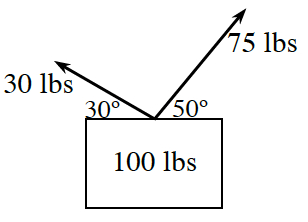### Home > PC3 > Chapter Ch8 > Lesson 8.3.3 > Problem8-121

8-121.

Two forces are being used to lift a $100$ pound box as shown in the diagram below. Can they lift the box? Use vector operations to justify your answer.

There are two forces pulling up on the box, but there is also the force of the box pulling straight down.
Compute the resultant force. Is it in a positive or negative vertical direction?

$\langle 30\cos\left(30^{\large \circ }\right), 30\sin\left(30^{\large \circ }\right)\rangle+\langle75\cos\left(50^{\large \circ }\right), 75\sin\left(50^{\large \circ }\right)\rangle+\langle0,-100\rangle$